# Creating a Spark job using Pyspark and executing it in AWS EMR

## Spark is considered as one of the data processing engine which is preferable, for usage in a vast range of situations. Data Scientists and application developers integrate Spark into their own…

### What is Spark?

Spark is considered as one of the data processing engine which is preferable, for usage in a vast range of situations. Data Scientists and application developers integrate Spark into their own implementations in order to transform, analyze and query data at a larger scale. Functions which are most related with Spark, contain collective queries over huge data sets, machine learning problems and processing of streaming data from various sources.

### What is PySpark?

PySpark is considered as the interface which provides access to Spark using the Python programming language. PySpark is basically a Python API for Spark.

### What is EMR?

Amazon Elastic MapReduce, as known as EMR is an Amazon Web Services mechanism for big data analysis and processing. This is established based on Apache Hadoop, which is known as a Java based programming framework which assists the processing of huge data sets in a distributed computing environment. EMR also manages a vast group of big data use cases, such as bioinformatics, scientific simulation, machine learning and data transformations.

#### Flowchart of the above functionalities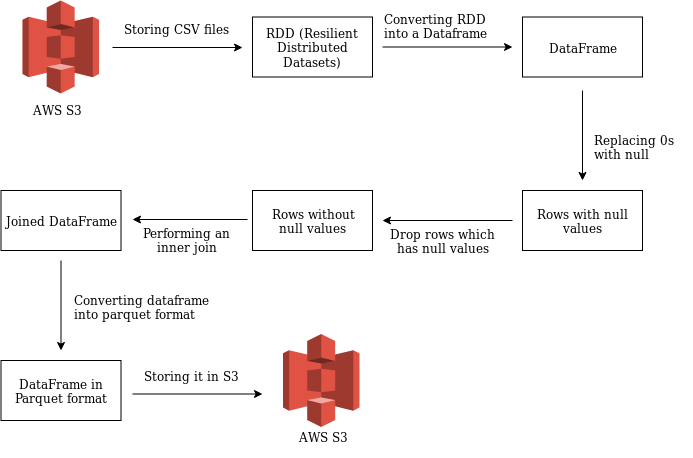Let me explain each one of the above by providing the appropriate snippets.

I've been mingling around with Pyspark, for the last few days and I was able to built a simple spark application and execute it as a step in an AWS EMR cluster. The following functionalities were covered within this use-case:

1. Reading csv files from `AWS S3` and storing them in two different RDDs (Resilient Distributed Datasets).
2. Converting an RDD into a Data-frame.
3. Replacing 0's with null values.
4. Dropping the rows which has null values.
5. Performing an inner join based on a column.
6. Saving the joined dataframe in the parquet format, back to S3.
7. Executing the script in an EMR cluster as a step via CLI.

Let me explain each one of the above by providing the appropriate snippets.

#### 1.0 Reading csv files from AWS S3:

This is where, two files from an S3 bucket are being retrieved and will be stored into two data-frames individually.

``````#importing necessary libaries
from pyspark import SparkContext
from pyspark.sql import SparkSession
from pyspark.sql.functions import *
from pyspark.sql.types import StringType
from pyspark import SQLContext
from itertools import islice
from pyspark.sql.functions import col

#creating the context
sqlContext = SQLContext(sc)

#reading the first csv file and store it in an RDD
rdd1= sc.textFile("s3n://pyspark-test-kula/test.csv").map(lambda line: line.split(","))

#removing the first row as it contains the header
rdd1 = rdd1.mapPartitionsWithIndex(
lambda idx, it: islice(it, 1, None) if idx == 0 else it
)
``````

#### 2.0 Converting an RDD (Resilient Distributed Datasets) into a Dataframe.

``````#converting the RDD into a dataframe
df1 = rdd1.toDF(['policyID','statecode','county','eq_site_limit'])

#print the dataframe
df1.show()
``````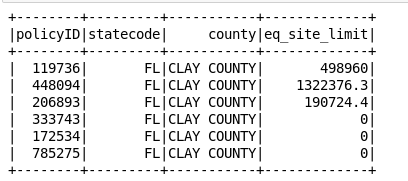#### 3.0 Replacing 0's with null values.

``````#dataframe which holds rows after replacing the 0's into null
targetDf = df1.withColumn("eq_site_limit",
when(df1["eq_site_limit"] == 0, 'null').otherwise(df1["eq_site_limit"]))

targetDf.show()
``````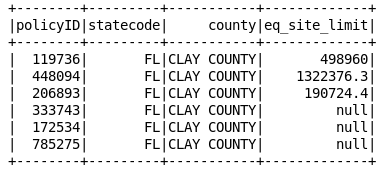#### 4.0 Dropping the rows which has null values.

``````df1WithoutNullVal = targetDf.filter(targetDf.eq_site_limit != 'null')
df1WithoutNullVal.show()
``````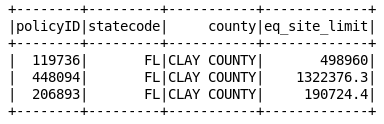#### Creating the second dataframe:

``````rdd2 = sc.textFile("s3n://pyspark-test-kula/test2.csv").map(lambda line: line.split(","))

rdd2 = rdd2.mapPartitionsWithIndex(
lambda idx, it: islice(it, 1, None) if idx == 0 else it
)

df2 = df2.toDF(['policyID','zip','region','state'])

df2.show()
``````

#### 5.0 Performing an inner join on both Dataframes:

``````innerjoineddf = df1WithoutNullVal.alias('a').join(df2.alias('b'),col('b.policyID') == col('a.policyID')).select([col('a.'+xx) for xx in a.columns] + [col('b.zip'),col('b.region'), col('b.state')])

innerjoineddf.show()
``````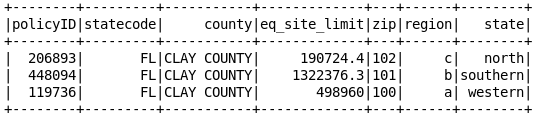#### 6.0 Saving the joined dataframe in the parquet format, back to S3.

``````innerjoineddf.write.parquet("s3n://pyspark-transformed-kula/test.parquet")
``````

Once we're done with the above steps, we've successfully created the working python script which retrieves two csv files, store them in different dataframes and then merge both of them into one, based on some common column.

#### 7.0 Executing the script in an EMR cluster as a step via CLI.

There after we can submit this Spark Job in an EMR cluster as a step. So to do that the following steps must be followed:

1. Create an EMR cluster, which includes Spark, in the appropriate region.
2. Once the cluster is in the WAITING state, add the python script as a step.
3. Then execute this command from your CLI (Ref from the doc) :
``````aws emr add-steps --- cluster-id j-3H6EATEWWRWS --- steps Type=spark,Name=ParquetConversion,Args=[ --- deploy-mode,cluster, --- master,yarn, --- conf,spark.yarn.submit.waitAppCompletion=true,s3a://test/script/pyspark.py],ActionOnFailure=CONTINUE
``````

If the above script has been executed successfully, it should start the step in the EMR cluster which you have mentioned. Normally it takes few minutes to produce a result, whether it's a success or a failure. If it's a failure, you can probably debug the logs, and see where you're going wrong. Otherwise you've achieved your end goal.

Complete source-code:

https://gist.github.com/Kulasangar/61ea84ec1d76bc6da8df2797aabcc721

#### References:

https://docs.aws.amazon.com/emr/latest/ManagementGuide/emr-what-is-emr.html

http://www.ibmbigdatahub.com/blog/what-spark